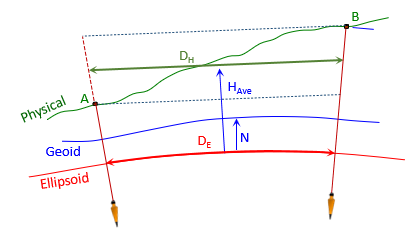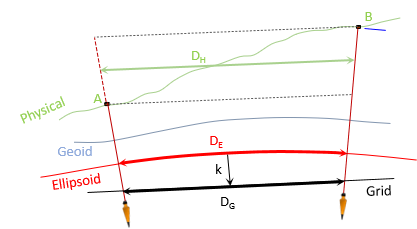### 2. Ground Distance to Grid

#### a. Ground to EllipsoidFigure L-2 Ground to Geodetic

We computed geodetic distances in Chapter K - those can be converted to meters to use here.
However, we'll do the computations from scratch using Equation H-2 to.Equation H-2
Jerry's -33.902 meter geoid height will be used as a project average.
##### (1) Geodetic distance Jerry-A1##### (2) Geodetic distance Jerry-B7#### b. Ellipsoid to GridFigure L-3 Geodetic to Grid

We'll use and compare the same reductions for UTM as we did for SPC. Table L-2 shows the grid scale at each point and how they were obtained.

 Table L-2 Grid Scales Point Scale Source A1 1.00020 6404 software Jerry-A1 midpoint 1.00020 9086 Equation H-4 Jerry 1.00021 177 datasheet Jerry-B7 midpoint 1.00021 5856 Equation H-4 B7 1.00020 8953 software

Jerry's CF from the datasheet is 1.00016 082.

Because the process is similar to SPC grid reduction, computations are not shown but results are summarized in Tables L-3 and L-4.

 Table L-3 Line Jerry-A1 Grid Reduction Comparisons DE x k k Grid Dist, m Diff, m Wtd Ave k 1.00020 9086 1305.3993 - - Average k 1.00020 9087 1305.3993 0.0000 Jerry's k 1.00021 177 1305.4028 +0.0035 Combined Factor DH x CF 1.00016 082 1305.4000 +0.0007

 Table L-4 Line Jerry-B7 Grid Reduction Comparisons DE x k k Grid Dist, m Diff, m Wtd Ave k 1.00021 4025 1568.8175 - - Average k 1.00021 0362 1568.8175 0.0000 Jerry's k 1.00021 177 1568.8111 -0.0064 Combined Factor DH x CF 1.00016 082 1568.8173 -0.0002
Unlike SPC reduction, using Jerry's scale as a project average resulted in the largest differences. Average line scale worked well. Jerry's CF reduction had small (inconsequential?) differences. Using CF reduction could be sufficient if the elevations of points A1 and B7 represent the range across the project.